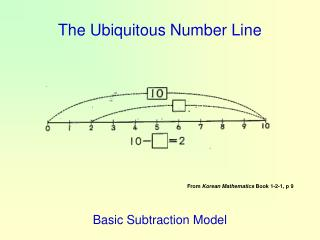DownloadDownload PresentationThe Ubiquitous Number Line

# The Ubiquitous Number Line

Télécharger la présentation## The Ubiquitous Number Line

- - - - - - - - - - - - - - - - - - - - - - - - - - - E N D - - - - - - - - - - - - - - - - - - - - - - - - - - -
##### Presentation Transcript

1. Basic Subtraction Model The Ubiquitous Number Line From Korean Mathematics Book 1-2-1, p 9

2. Comparison Subtraction Model The Ubiquitous Number Line From Korean Mathematics Book 1-1-5 pg 65

3. Time modeled on Number Line The Ubiquitous Number Line the time of the time of starting cleaning finishing cleaning From Korean Mathematics Book 2-1-6, pg 62

4. The Ubiquitous Number Line Fill in the box with a fraction or decimal. From Korean Mathematics Book 3-2-7, p 95 Comparison of Fractions with Decimals

5. The Ubiquitous Number Line Let’s find a way to add the numbers 0.5 and 0.3. 0.5 is 5 of 0.1 and 0.3 is 3 of 0.1. The sum of 0.5 and 0.3 is 8 of 0.1. 0.5 + 0.3 = 0.8 From Korean Mathematics Book 3-2-7, p 96 Addition of Decimals

6. The Ubiquitous Number Line Let’s think of finding the difference between 0.56 and 0.32. From Korean Mathematics Book 4-2-6, p 78 Subtraction of Decimals

7. The Ubiquitous Number Line Using the number line above, From Korean Mathematics Book 4-2-6, p 78 Subtraction of Decimals

8. Division of Whole into Fractions on Number Line The Ubiquitous Number Line Stanford is making two animal shapes by dividing in half a wire 5 m in length. Let’s find out the length of the wire for one animal shape. How can we do the division, 5 ÷ 2? From Korean Mathematics Book 5-2-2, pg 26

9. There are 45 children in the class. enjoy physical education. of the remainder like math. How many like math? 45 students From Korean Mathematics Book 6-2-9, p 133 Three Step, Mixed Operation

10. Leads to Equations A stack of wood is 40.5 cm high. Among them are 15 boards which are 0.9 cm thick. The rest are 1.5 cm thick. How many 1.5 cm thick boards are there? Identification of x (0.9 × 15) + (1.5 × x) = 40.5 From Korean Mathematics Book 6-2-9, p 135